Games
Problems
Go Pro!

# Calculus

Lesson Plans > Mathematics > Calculus

# Featured Lesson Plans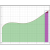Introducing students to the Fundamental Theorem of Calculus, using a bit of geometry to provide a basic proof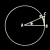A unique way of looking at the Squeeze Theorem, and an application of the theorem in trigonometry - Two Policemen and a Drunk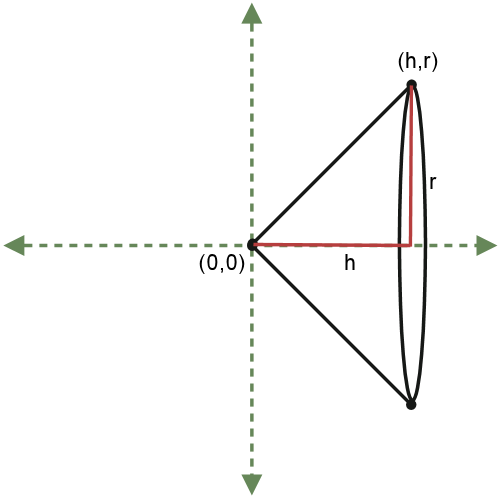Solids of revolution: Calculus lesson involving a proof of the volume of a cone formula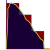A calculus lesson involving hiking, hiking poles, weight distribution, and the area under the curve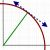Using a circle to help illustrate slopes of curved lines; use progressive approximations, and an exact value using geometric principles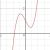A slide show with a series of simple real-world maximum and minimum problems that can be solved using calculus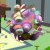Show students the importance of being able to find the slope of a curved line, using...a video game?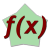Worksheet involving taking derivatives of products and quotients of functions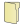Like us on Facebook to get updates about new resources